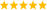# LabVIEW

cancel
Showing results for
Did you mean:

Solved!
Go to solution

## function fit with a variable number of cubic curves

Hi,

I have a set of data&colon; a set representing the independent variable and a set representing the dependent variable. I need to find the minimum number of cubic curves (find their coefficients) that reduce the mean square error less than a given tollerance. If the tollerance is higher than given one with 17 segments, then I need to throw an error.

Have you an idea which function blocks I need of?

I could use General Polynomial Fit VI with order 3 and its default method to check the residual. If the residual is higher than the given tollerance, then I could try to use two cubic curves and test them on different starting and ending points until the residual is less than the given tollerance. If the tollerance is not guaranteed, I add another curve and I test again the fitting as above. Iteratively until the residual is less than the given tollerance or until I would need to add the 18th cubic curve.

If there were something ready, I would appreciate.

Thanks.

Message 1 of 10
(1,439 Views)

## Re: function fit with a variable number of cubic curves

Can you clarify a bit more about continuity and smoothness at the boundary between adjacent cubics?  Are the individual cubics required to meet at the same point?  Are the first derivatives required to be the same at the boundary?

-Jim

Message 2 of 10
(1,408 Views)

## Re: function fit with a variable number of cubic curves

Maybe you could use cubic spline fit and look at the residual as a function of the e.g. the balance parameter.LabVIEW Champion. It all comes together inGCentralMessage 3 of 10
(1,397 Views)

## Re: function fit with a variable number of cubic curves

The cubics are required to meet at the same point, but the first derivative can be discontinued. So the spline is not required.

My final algorithm is that:

1) Find first cubic curve with General Polynomial Fit VI, inserting 5 points [X,Y].

2) Subtract calculated Y from real Y to find error. If the absolute value of the error is greater than tollerance or there aren't more point, then go to step 3), in the other case, return to step 1 inserting a new point after the points already used.

3) Save the coefficient of the curve calculated on step 2, then reject all the points used with exception of the last point that cause the error greater than the tollerance. If there are more points and the curves calculated are less than 17, then return to point 1, in the other case exit.

There is a better way? Where I can find the explanation of the fitting algorithms?

Thanks.

Message 4 of 10
(1,344 Views)

## Re: function fit with a variable number of cubic curves

There is some numerical error in Labview Fitting Libraries, so I decide to create my own algorithm.

There is the algorithm attached.

The result is wrong. What is wrong in my VI? With four fitting points the numerical error should be very very low

Here the theory behind:

Message 5 of 10
(1,285 Views)

## Re: function fit with a variable number of cubic curves

@Fabiobonelli wrote:

There is some numerical error in Labview Fitting Libraries,

Can you be more specific?

Here are two possible alternatives using LabVIEW primitives. Seems to give the correct result.LabVIEW Champion. It all comes together inGCentralMessage 6 of 10
(1,271 Views)

## Re: function fit with a variable number of cubic curves

Also, your x-range is far from zero and over a relatively narrow range. This can cause problems with conditioning. Your data is probably OK, but this is something to consider if the data is even more extreme. You probably would get more accuracy of you would re-center on an average x value and correct for the shift later.LabVIEW Champion. It all comes together inGCentralMessage 7 of 10
(1,261 Views)

## Re: function fit with a variable number of cubic curves

X = -6453

Y = -266

Check the residuals.

Thanks.

Message 8 of 10
(1,236 Views)
Solution
Accepted by topic author FabioBone

## Re: function fit with a variable number of cubic curves

@Fabiobonelli wrote:

X = -6453

Y = -266

Check the residuals.

Thanks.

Did you even read my reply? You have a serious conditioning problem because the data is over a narrow range far from zero. For example -6500^3 is smaller than -2E11, i.e. a value that is many (many!) orders of magnitude different than the constant term (1). The resulting linear algebra problem is very ill conditioned and just blindly stepping on the gas pedal is ill adviced here.

This is not a problem with the LabVIEW implementation, but a fundamental flaw that you would encouter equally (or even worse) if you would do your own math. No way around it. (See also)

My recommendation was to remove the offset from X and add it later again. Now things work correctly. (note that fitting to a second order polynomial still works without the tweak).

Try the attached and see for yourself.LabVIEW Champion. It all comes together inGCentralMessage 9 of 10
(1,211 Views)

## Re: function fit with a variable number of cubic curves

I haven't got your warning. Using reconditioning offset, my algorithm works very well. I was searching how Labview fits the data and I didn't catch the error in input data.

Thank you.

Message 10 of 10
(1,192 Views)